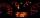# Edges or sides

Calculate the cube volume, if the sum of the lengths of all sides is 276 cm.

Result

V =  12167 cm3

#### Solution:Leave us a comment of example and its solution (i.e. if it is still somewhat unclear...):Be the first to comment!#### To solve this verbal math problem are needed these knowledge from mathematics:

Tip: Our volume units converter will help you with converion of volume units.

## Next similar examples:

1. Cube edgesThe sum of the lengths of the cube edges is 42 cm. Calculate the surface of the cube.
2. Third dimensionCalculate the third dimension of the cuboid: a) V = 224 m3, a = 7 m, b = 4 m b) V = 216 dm3, a = 9 dm, c = 4 dm
3. Diameter of a cylinderI need to calculate the cylinder volume with a height of 50 cm and a diameter of 30 cm.
4. The cubeThe cube has an edge of 25 cm. We cut it into small cubes of 5 cm long side. How many of these little ones left when we build a new cube of 20 cm in length?
5. Cube basicsHow long is the edge length of a cube with volume 23 m3?
6. Cube 3How many times will increase the volume of a cube if we double the length of its edge?
7. Cube cornersThe wooden cube with edge 64 cm was cut in 3 corners of cube with edge 4 cm. How many cubes of edge 4 cm can be even cut?
8. Volume of cubeSolve the volume of a cube with width 26cm .
9. The numberThe number of 1 cm cubes required to make 4 cm cube is?
10. AquariumAquarium is cube with edge 45 cm. How much water can fit in there?
11. A cylindrical tankA cylindrical tank can hold 44 cubic meters of water. If the radius of the tank is 3.5 meters, how high is the tank?
12. Fire tankHow deep is the fire tank with the dimensions of the bottom 7m and 12m, when filled with 420 m3 of water?
13. Car consumptionThe car has passed 988 kilometers and consumed 66 liters of petrol. What is the consumption per 100 kilometers?
14. ExpressionSolve for a specified variable: P=a+4b+3c, for a
15. SimplifySimplify the following problem and express as a decimal: 5.68-[5-(2.69+5.65-3.89) /0.5]
16. HotelThe hotel has a p floors each floor has i rooms from which the third are single and the others are double. Represents the number of beds in hotel.
17. AlleyAlley measured a meters. At the beginning and end are planted poplar. How many we must plant poplars to get the distance between the poplars 15 meters?